# Single-Phase, Grid-Connected PV Inverter with Partial Shading (Equation-Based PV Cell, P&O and dP/dV MPPT)

This PLECS demo model illustrates a grid-connected solar panel system with a boosted front end and a single-phase inverter back end. The boost converter is designed to operate the panel at its maximum power point (MPP). A maximum power point tracking (MPPT) algorithm is implemented to improve the performance of the solar panel under partial shading conditions. Further, the inverter is operated with an outer voltage loop to control the DC-link voltage and a synchronous regulator to maintain unity power factor.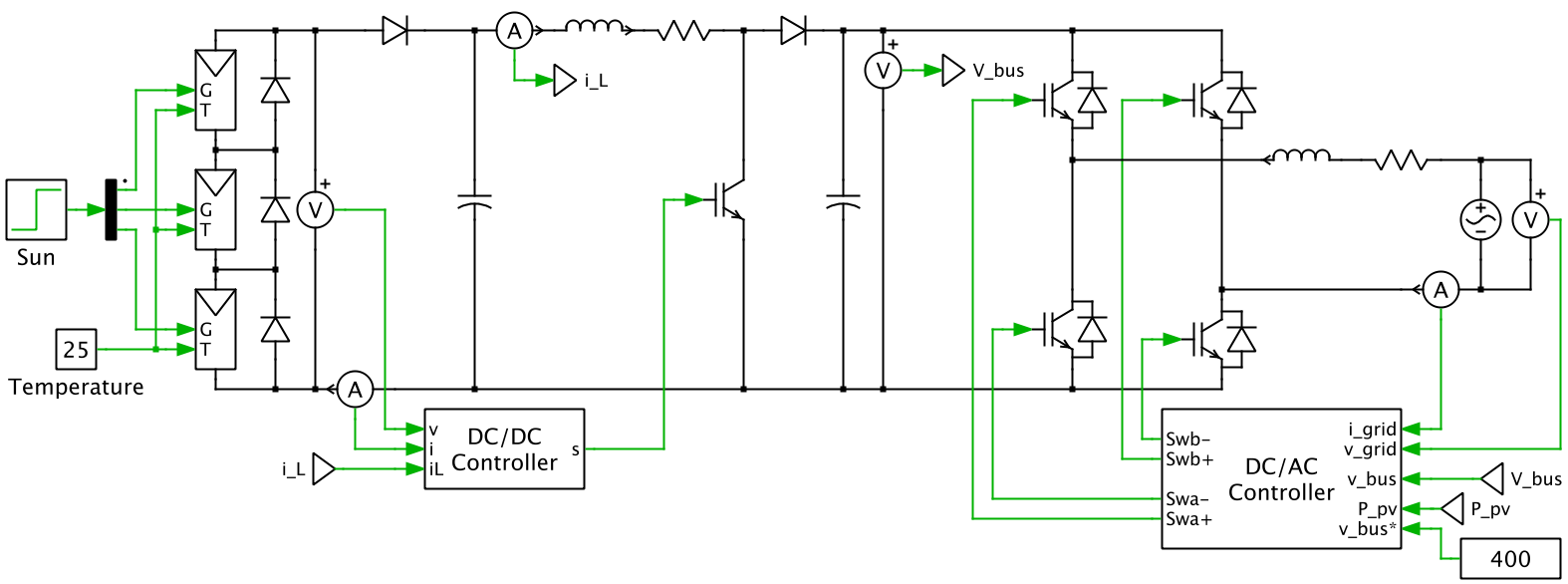### Photovoltaic system model

An equivalent single-diode model is used to implement the PV substring. The equation governing the current generated by the unit is given by:

Im = IPV - I0 {e[(V + RsI) / (Vta)] - 1}

where Ipv is the current generated by the light incident on the unit, I0 is the reverse saturation or leakage current of the diode, V is the terminal voltage, Rs is the equivalent series resistance, I is the terminal current, Vt is the thermal voltage, and a is the diode ideality factor.

The PV module consists of three of these substrings connected in series. Each substring has a bypass diode. The solar irradiance (input G) and temperature (input T) are assumed to be uniform across each substring. In the case of partial shading, the irradiance on each substring is unequal, decreasing the magnitude of the current generated by the shaded substring. In the absence of the bypass diode, this can cause reverse current and power dissipation in the shaded cells. The bypass diode allows the difference between the current generated by the shaded and unshaded substrings to flow through the bypass diode and avoids reverse current and power dissipation in the shaded cells.

The PV units modeled in this simulation correspond to an array of three parallel-connected KC200GT solar modules, with 54 series-connected solar cells in each KC200GT module.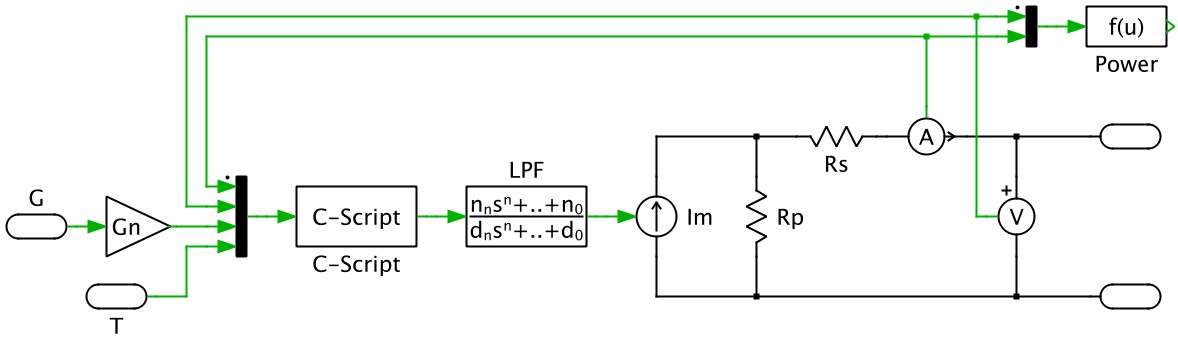### DC/DC converter

A boost converter is controlled to operate the solar panel at the MPP. Two MPPT algorithms are implemented: Perturb and Observe (P&O) and Incremental Conductance (INC). The P&O or INC algorithm can be selected from "MPP controller" in the "DC/DC Controller" subsystem.

These MPPT algorithms change the reference voltages to increase the output power of the solar panel. As the algorithm changes the reference voltage beyond the MPPT, power decreases. This decrease in power is detected and the reference voltage is changed in the opposite direction.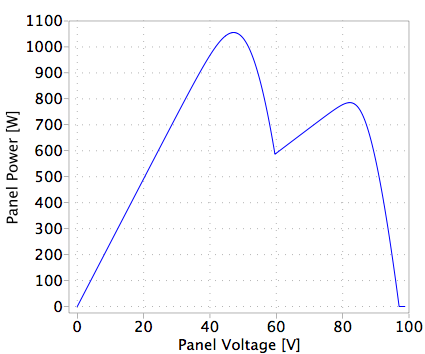In partial shading conditions, multiple local maximum power points (LMPP) occur, as can be seen in the plot above. These LMPPs are a result of the bypass diodes in the PV module. If a single-stage MPPT algorithm is employed, depending on the starting voltage reference, the controller might get trapped in a LMPPs that is not the global maximum power point (GMPP). A second stage is required to operate the converter at the GMPPT. A voltage scan is performed across the entire voltage range for the PV system and the voltage at which maximum power is produced is recorded. This voltage range scan can be enabled as the second stage for the controller to operate the converter at the GMPPT.

The DC/DC controller comprises of a three level, nested-loop structure. The MPP controller forms the outer loop and generates the voltage setpoint for the voltage controller. The voltage loop is used to control the input capacitor voltage of the boost converter. The voltage loop generates a current setpoint for the inner current loop. This current regulator is used to regulate the inductor current. The output of the current loop is used to derive the duty cycle for the boost converter.

### DC/AC converter

The output of the boost converter is connected to the DC-side of a single-phase voltage source inverter (VSI) via a DC-link capacitor. The VSI is regulated by a nested control scheme with an outer voltage loop and an inner current loop.

The outer voltage loop is used to control the DC-link voltage and maintain the voltage at the desired level. The voltage loop generates the grid current amplitude setpoint. Since the converter is operated with zero reactive power, the generated current setpoint from the voltage controller is equal to the setpoint of the q-axis current in the synchronous frame regulator.

The synchronous frame regulator reduces the complexity by transforming the AC system to an equivalent DC system and then implementing PI controllers for the d- and q-axis, respectively. In single-phase systems, unlike three-phase systems, not enough information is available to directly convert the AC current into the synchronous frame. In this simulation, the Fictive Axis Emulation (FAE) approach is implemented. The grid current is assumed to be in line with the α-axis, and the β-axis currents are emulated using the FAE. The measured α and derived β currents are transformed into the dq-axis and fed into the synchronous-frame regulator. The angle used for the transformation is generated by a phase lock loop (PLL).

The synchronous frame regulator calculates a desired αβ voltage vector setpoint. This vector is scaled by the peak grid voltage amplitude to generate the modulation index for the modulator. The modulation indices are used to generate the switching signals for the VSI using a unipolar modulator. The control structure for the DC/AC converter is shown below.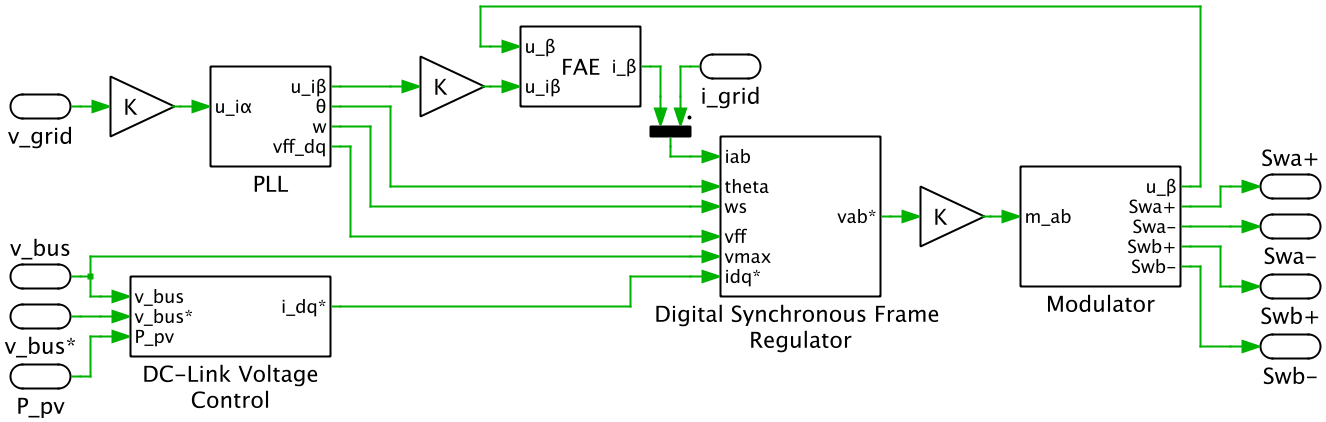### Simulation

The simulation demonstrates the operation of a grid-tied solar inverter operated at its MPP. The control system is initially disabled and the brakers remain open. Once the PLL has locked, all control systems are enabled and the brakers are closed. The reference voltage is changed resulting in a rise in the power generated by the solar panel until the MPP is reached. The panels are initially unshaded, but at 0.7 sec, Substring 3 experiences partial shading. The irradiance decreases from 100% to 65% of the nominal value (1000 W/m2). The GMPP search is enabled and the controller quickly reaches the global maximum.

The grid-side controller maintains the DC-link capacitor voltage at the desired 400 VDC. It also maintaining unity power factor and delivers power generated by the solar panels to the grid. The figure below shows the grid side voltage, current, and power when the system is operating with no partial shading.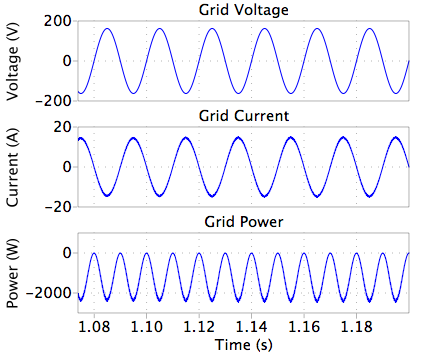### Try it

This model is available in the PLECS Demo Model library provided in both versions of PLECS.

Categories: